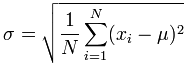# Standard Deviation Calculator

Here are the step-by-step calculations to work out the Standard Deviation (see below for formulas).

Enter your numbers below, the answer is calculated "live":

 When your data is the whole population the formula is: (The "Population Standard Deviation")When your data is a sample the formula is: (The "Sample Standard Deviation")The important difference is "N-1" instead of "N" ... read more at Standard Deviation Formulas.